# 人教版小學數學二年級上冊期中檢測卷及答案

1.我們用的數學課本上有（ ）個角，它們都是（ ）角。

2.把直角、銳角、鈍角按照從小往大的順序排列是（ ）<（ ）<（ ）。

3.下圖中有（ ）個直角。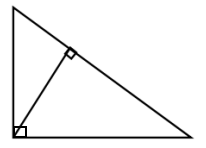4.5×6=30，在這道乘法算中，（ ）和（ ）是乘數，（ ）是積，讀作（ ），運用到的乘法口訣是（ ）。

5.已知相同的加數是2，相同加數的個數是5，我們可以根據這句話寫出兩個乘法算式是（ ）或（ ），給它的結果再加上87，和是（ ）。

6.在下面的（ ）里填上“<”“>”或“=”。

2米（ ）1米+100厘米 40厘米（ ）1米

56厘米（ ）2米-200厘米 4米（ ）55厘米

100-58（ ）57+39 40+29（ ）100

91-18（ ）100-21 38+29-10（ ）50

1.下圖中有2條線段。 （ ）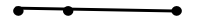2.用2根小棒可以擺出4個直角。 （ ）

3.角有一個頂點和一條邊。（ ）

4.3×2=6，讀作：2乘3等于6。 （ ）

5.4×6=6+6+6+6 （ ）

1. 小明家里的書架子上原本放著72本書，上周借出去了17本，還剩下多少本書？下面列式正確的是（ ）。

A.72-17 B.72+17 C.17+72

2.二年級一班有男生28人，女生22人。______________？可以提出的問題是（ ）。

A.女生比男生多多少人

B.男生比女生多多少人

C.二年級一班比二年級二班多多少人

3.角的大小跟（ ）有關系。

A.角的位置

B.角的兩條邊的長短

C.角的張口的大小

4. 下面的等式中，正確的是（ ）。

A.4×5=4+5

B.4×5=5+5+5

C.4×5=4+4+4+4+4

5.表示4個3相加的算式是（ ）。

A.4+3 B.4×3 C.4-3

87-34= 100-71=

44+28= 90-22=

23+59= 29+61-33=

99-34+25= 77+23-46=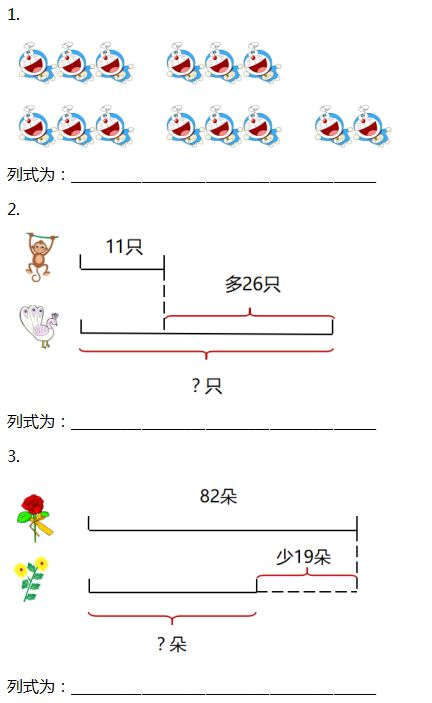1.有兩根長都是48厘米的木棍，把它們粘在一起后長度為80厘米，粘在一起的長度是多少厘米？（10分）

2.下午的時候有多少人在繪畫展覽廳參觀？（9分）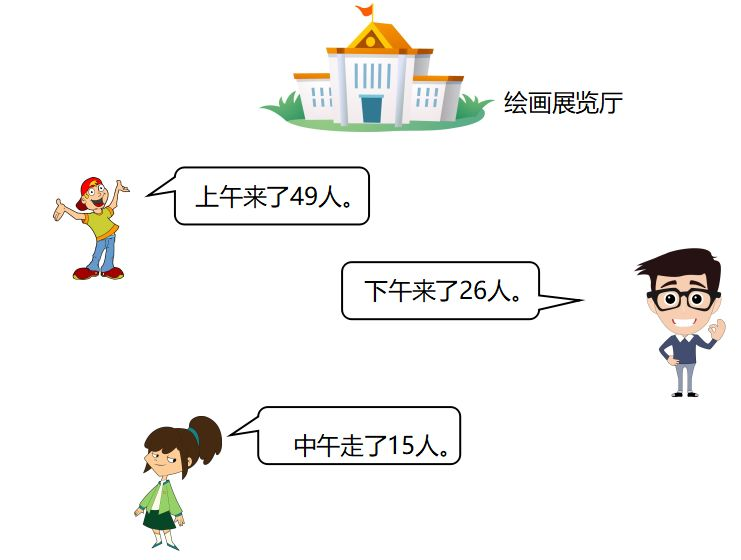3.小明的書放在書架上，放5堆，每堆3本書，還剩2本；小紅的書也放在書架上，放6堆，每堆4本，還差2本。（9分）

（1）小明一共有多少本書？

（2）小紅一共有多少本書？

（3）小紅和小明共有多少本書？

1.4，直角

2.銳角，直角，鈍角

3.3

4.5,6,30,5×6=30,五六三十

5.2×5=10,5×2=10,97

6.

2米（ = ）1米+100厘米 40厘米（ < ）1米

56厘米（ > ）2米-200厘米 4米（ > ）55厘米

100-58（ < ）57+39 40+29（ < ）100

91-18（ < ）100-21 38+29-10（ > ）50

× √ × × √

A B C C B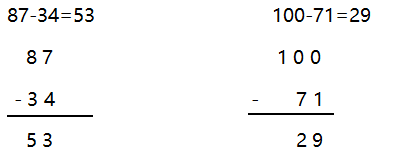1.3×4+2=14

2.11+26=37（只）

3.82-19=63（朵）

1.48+48-80=16（厘米）

2.49+26-15=60（人）

3.（1）5×3+2=17（本）

（2）6×4-2=22（本）

（3）17+22=39（本）

###熱門文章推薦95.6%的用戶看完本文后，還關注了以下精彩公眾號